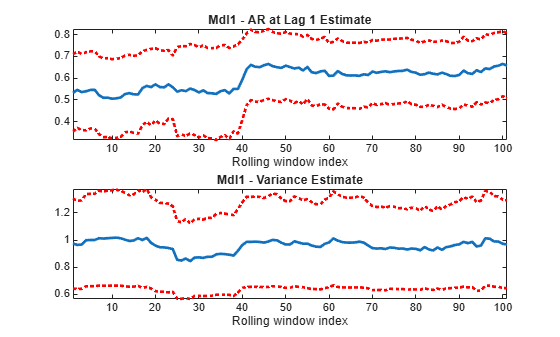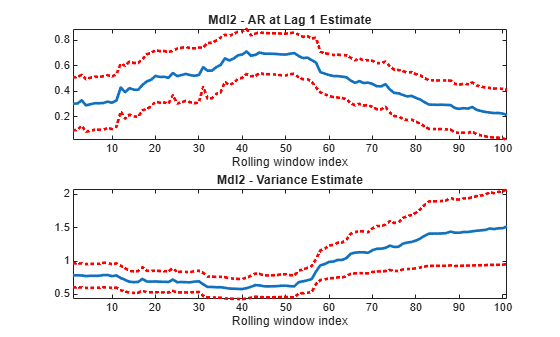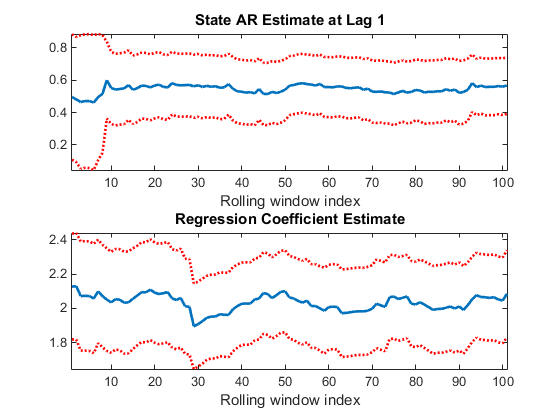Main Content

## Assess State-Space Model Stability Using Rolling Window Analysis

### Assess Model Stability Using Rolling Window Analysis

This example shows how to use a rolling window to check whether the parameters of a time-series model are time invariant. This example analyzes two time series:

• Time-series 1: simulated data from a known, time-invariant model

• Time-series 2: simulated data from a known, time-varying model

Completely specify this AR(1) model for Time-series 1:

`${y}_{t}=0.6{y}_{t-1}+{\epsilon }_{t}$`

where ${\epsilon }_{t}$ is Gaussian with mean 0 and variance 1. Completely specify this time-varying model for Time-series 2:

`$\begin{array}{c}{y}_{t}=0.2{y}_{t-1}+{\epsilon }_{t};t=1,...,100\\ {y}_{t}=0.75{y}_{t-1}+{\epsilon }_{t};t=101,...,150\\ {y}_{t}=-0.5{y}_{t-1}+{\epsilon }_{t};t=151,...,200,\end{array}$`

where ${\epsilon }_{t}$ is Gaussian with mean 0 and variance 1.

```Mdl1 = arima('AR',0.6,'Constant',0,'Variance',1); Mdl2 = cell(3,1); % Preallocate ARMdl2 = [0.2 0.75 -0.5]; for j = 1:3 Mdl2{j} = arima('AR',ARMdl2(j),'Constant',0,'Variance',1); end```

`Mdl1` is an `arima` model objects. You can access its properties using dot notation. `Mdl2` is a cell array of `arima` model objects. You can you cell indexing and dot notation to access properties of the models within `Mdl2`. For example, to access the AR parameter value of the third model in `Mdl3`, enter `Mdl2{3}.AR`.

Simulate `T` = 200 periods of data from `Mdl1` and `Mdl2`. Use a presample response of 0 for both series.

```rng(1); % For reproducibility T = 200; y1 = simulate(Mdl1,T,'Y0',0); timeMdl2 = [100 50 50]; % Number of observations per model in Mdl2 y2 = 0; for k = 1:numel(Mdl2) y2 = [y2; simulate(Mdl2{k},timeMdl2(k),'Y0',y2(end))]; end Y = [y1 y2(2:end)];```

Specify empty AR(1) models for the estimation of `Mdl1`, `Mdl2`, and `Mdl3`. Estimate all three models using the respective data sets and a rolling window size of 40 periods. Also, use a rolling window increment of one period. Store the autoregressive parameters and estimated innovations variance.

```Mdl = arima('ARLags',1,'Constant',0); m = 100; % Rolling window size N = T - m + 1; % Number of rolling windows EstParams = cell(2,1); % Preallocate for estimates EstParamsMat = zeros(N,2); EstParamsSE = cell(2,1); EstParamsSEMat = zeros(N,2); for j = 1:2 for k = 1:N idxRW = k:(m + k - 1); % In-sample indices [EstMdl,EstParamCov] = estimate(Mdl,Y(idxRW,j),'Display','off'); EstParamsMat(k,:) = [EstMdl.AR{1} EstMdl.Variance]; EstParamsSEMat(k,:) = sqrt([EstParamCov(2,2) EstParamCov(3,3)]); end EstParams{j} = EstParamsMat; EstParamsSE{j} = EstParamsSEMat; end```

Plot the estimates and their point-wise confidence intervals over the rolling window index.

```titleMdls = {'Mdl1','Mdl2'}; for j = 1:2 figure; subplot(2,1,1); Estimates = EstParams{j}; SEs = EstParamsSE{j}; plot(Estimates(:,1),'LineWidth',2); hold on; plot(Estimates(:,1) + 2*SEs(:,1),'r:','LineWidth',2); plot(Estimates(:,1) - 2*SEs(:,1),'r:','LineWidth',2); title(sprintf('%s - AR at Lag 1 Estimate',titleMdls{j})); xlabel 'Rolling window index'; axis tight; hold off; subplot(2,1,2); plot(Estimates(:,2),'LineWidth',2); hold on; plot(Estimates (:,2) + 2*SEs(:,2),'r:','LineWidth',2); plot(Estimates(:,2) - 2*SEs(:,2),'r:','LineWidth',2); title(sprintf('%s - Variance Estimate',titleMdls{j})); xlabel 'Rolling window index'; axis tight; hold off; end```For `Mdl1`, the AR estimate does not vary much from 0.6, and the estimates are not significantly different from one another (pair-wise). Similar results occur for the variance of `Mdl1`. The AR estimate of `Mdl2` grows, and then falls, which indicates time dependence. Also, based on the confidence intervals, there is evidence that some estimates differ from others. Though the variance did not change during simulation, there seems to be heteroscedasticity possibly induced by the instability of the model.

### Assess Stability of Implicitly Created State-Space Model

This example shows how to specify and estimate a state space model when conducting a rolling window analysis for stability. A rolling window analysis for an explicitly defined state-space model is straightforward, so this example focuses on implicitly defined state-space models.

Consider this state-space model:

`$\begin{array}{c}{x}_{t}=\varphi {x}_{t-1}+{\epsilon }_{t}\\ {y}_{t}-\beta {z}_{t}={x}_{t}+{u}_{t}\end{array},$`

where εt and ut are Gaussian process with mean 0 and variance 1. Create the function `rwParamMap.m`, which specifies how the parameters in `params` map to the state-space model matrices, the initial state values, and the type of state, and save it in your working folder.

```function [A,B,C,D,Mean0,Cov0,StateType,deflateY] = rwParamMap(params,y,Z) %rwParamMap Parameter-to-matrix mapping function for rolling window example %using ssm and specifying an AR(1) state model % The state space model specified by rwParamMap contains a stationary % AR(1) state, the observation model includes a regression component, and % the variances of the innovation and disturbances are 1. The response y % is deflated by the regression component specified by the predictor % variables x. A = params(1); B = 1; C = 1; D = 1; Mean0 = []; Cov0 = []; StateType = 0; deflateY = y - params(2)*Z; end ```

The software does not support the simulation of implicit models containing a regression component. Therefore, to simulate data from this model, you must specify all model components up to the regression component. You can do this explicitly since this example uses a simple state-space model. Otherwise, you can create another function and define another state-space model implicitly (e.g., for time-varying state-space models).

`Mdl2Sim = ssm(NaN,1,1,1,'StateType',1);`

`Mdl2Sim` is an implicitly defined `ssm` object.

Simulate a 200-period path of random standard Gaussian data. Then, simulate responses from `Mdl2Sim`, and inflate the responses with the regression component. For this example, use ϕ = 0.6 and β = 2.

```rng(1); % For reproducibility T = 200; Z = randn(T,1); phi = 0.6; beta = 2; deflateY = simulate(Mdl2Sim,T,'Params',phi); y = deflateY + Z*beta;```

`y` is the inflated, simulated response path, and `Z` is the simulated predictor series.

If you define a state-space model implicitly and the response and predictor data (i.e., `y` and `Z`) exist in the MATLAB® Workspace, then the software creates a link from the parameter-to-matrix mapping function those series. If the data do not exist in the MATLAB Workspace, then the software creates the model, but you must provide the data using the appropriate name-value pair arguments when you, e.g., estimate the model.

Therefore, to conduct a rolling window analysis when the state-space model is implicitly defined and there is a regression component, you must specify the state-space model indicating the indices of the data to be analyzed for each window. Conduct a rolling window analysis of the simulated data. Let the rolling window length be 100 periods for this example.

```m = 100; N = T - m + 1; % Number of rolling windows EstParams = nan(N,2); % Preallocation EstParamSE = nan(N,2); for j = 1:N; idxRW = j:(m + j - 1); Mdl = ssm(@(c)rwParamMap(c,y(idxRW),Z(idxRW))); [~,EstParams(j,:),EstParamCov] = estimate(Mdl,y(idxRW),[0.5 1]',... 'Display','off'); EstParamSE(j,:) = sqrt(diag(EstParamCov)); end```

Plot the estimates and point-wise confidence intervals for the AR parameter and regression coefficient.

```figure; subplot(2,1,1); plot(EstParams(:,1),'LineWidth',2); hold on; plot(EstParams(:,1) + 2*EstParamSE(:,1),':r','LineWidth',2); plot(EstParams(:,1) - 2*EstParamSE(:,1),':r','LineWidth',2); title 'State AR Estimate at Lag 1'; xlabel 'Rolling window index'; axis tight; hold off; subplot(2,1,2); plot(EstParams(:,2),'LineWidth',2); hold on; plot(EstParams(:,2) + 2*EstParamSE(:,2),':r','LineWidth',2); plot(EstParams(:,2) - 2*EstParamSE(:,2),':r','LineWidth',2); title 'Regression Coefficient Estimate'; xlabel 'Rolling window index'; axis tight; hold off;```The plots indicate that the model is stable since the AR estimate does not deviate much from its mean, nor does the regression coefficient estimate.

Download ebook